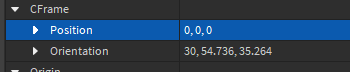# How does the new CFrame System work?

Hi, I just wanted to ask you if you can tell me why the code doesn’t work as desired because I want to learn when to use and how to use CoordinateFrames. My problem is that when I run this code (this is just a test code btw):
`workspace.Part.CFrame = CFrame.Angles(math.rad(45), math.rad(45), 0)` ,

I want it only to rotate and not to go to the origin. I don’t understand. I just used CFrame.Angles() to rotate and not CFrame.new(0,0,0).

I also looked at the docs and this post from a couple months ago: Improved CFrame support in Studio but I couldn’t find a solution.

I have no idea what is going on there and what math.rad() is and what CFrame generally is, I have only watched TheDevKings and AlvinBlox’s tutorials but these are probably outdated since they’re four years old

And what if I want to do it with a model? Should I use PrimaryPartCFrame? Because when I do `Model.CFrame` it doesn’t work anymore

And by the way: The orientation script didn’t even work:Hello, for you first question you can get the part position and multiply with the angles:

``````workspace.Part.CFrame = workspace.Part.CFrame * CFrame.Angles(math.rad(45), math.rad(45), 0)
``````

For the second question, yes, you have to get the Model PrimaryPart to move a model CFrame, but before doing that u have to make sure the model has a primary part. One way to move a model can be PivotTo().

1 Like

Ok thanks but can someone explain what CFrame is or link an updated tutorial? I still appreciate your answer but I don’t quite understand why I have to multiply it?

CFrame.Angles is for rotation value in a CFrame, and CFrame.new is for the position value. You cannot just use CFrame.Angles, since you didn’t define a position. To have a rotation and a position, you can use

``````local YourPositionVariable = workspace.Part.CFrame
``````
1 Like

You just need to multiply it for rotations. A simple tutorial: Roblox CFrames - Position and Rotate Objects (2022 Roblox Studio Tutorial Beginners Series) (B003) - YouTube

1 Like

Ok but why multiply it? Does that have anything to do with maths and why didn’t they just do 2 parameters

If you add the Position and the angles will result in an error. Multiplying changes the part orientation

1 Like

I’m honestly not sure, it’s probably to do with math otherwise I don’t see why they wouldn’t just do 2 parameters.

1 Like

Ok, we’ll just accept it haha. And roblox wants me to write at least ## characters and for some reason they don’t want me to write the count of characters

Hopefully one of the solutions listed here works! Make sure to mark it as the solution if it does btw

1 Like

Yeah but I don’t want to use radians because I’m more familiar with degrees. Is there a math.degree() function or do I just put the numbers in naked

put the numbers in by themselves and it should work.

1 Like

A CFrame value contains both an object’s positional and rotational values, so by doing `obj.CFrame = CFrame.Angles(...)` you override an object’s both positional and rotational value and replace them with only a rotational value. Hence the positional value is assumed to be zero.

2 Likes

Thanks man. I never understood why that is like that, but with your explanation I understand it!

This topic was automatically closed 14 days after the last reply. New replies are no longer allowed.